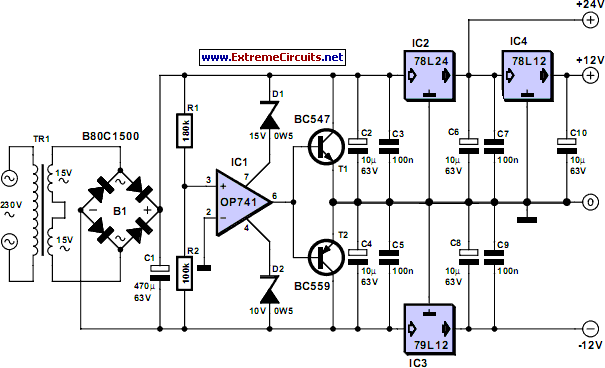# Learning Electronics

Learn to build electronic circuits

# Triple Power Supply

Inexpensive miniature transformers normally provide one or two secondary voltages, which is sufficient for generating a set of positive and negative supply voltages, such as are needed for operational amplifier circuits. But what can you do if you need an additional voltage that is higher than either of the supply voltages (such as a tuning voltage for a receiver?). This circuit shows a simple solution to this problem, and it certainly can be extended to suit other applications. Using a 2×15-V transformer, it generates positive 24-V and 12-V supply voltages and a negative 12-V supply voltage. The little trick for generating the +24-V output consists of using IC1 to create a virtual ground.

This is based on a well-known circuit with a voltage divider formed by two equal-valued resistors, which divide the voltage Ub across the rectifier from approximately 40 V down to 20 V. This Ub/2 potential is buffered by an opamp, which allows this virtual ground to drive a load. The present circuit uses the same principle, but instead of being divided by a factor of 2, the voltage across the rectifier (approximately 40 V) is divided unequally by R1 and R2. The resulting potential, which is buffered by the opamp and the subsequent transistor output stage, lies approximately 15 V above the lower potential, and thus around 25 V below the upper potential.

Circuit diagram:Triple Power Supply Circuit Diagram

The three voltages are stabilised using standard 100-mA voltage regulators, as shown in the schematic. The supply voltages for the opamp are also asymmetric. Thanks to the low current consumption, this can be managed using two Zener diodes. You must bear in mind that the secondary voltage generated by an unloaded miniature transformer is significantly higher than its rated secondary voltage. The following results were obtained in a test circuit using a 1.6-VA transformer with two 15-V secondary windings: the positive and negative 12-V outputs could be loaded at around 10 mA each, and the 24-V output could be loaded with approximately 20 mA, all without any drop in any of the output voltages. For small circuits such as a 0(4)–20-mA instrumentation loop, this is fully adequate. For more complex circuits or switched loads, additional compensation may be necessary.
Author: Bernd Schädler - Copyright: Elektor Electronics